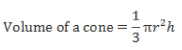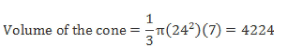A triangle is rotated about an axis which coi...
A triangle is rotated about an axis which coincides with one of its sides. If the sides of triangle are 7 cm, 24 cm and 25 cm, then what is the maximum possible volume(in cm3) that is generated in space by rotating the triangle?
A triangle is rotated about an axis which coincides with one of itssid...
Solution: 7, 24 and 25 is a Pythagorean triplet.When a triangle is rotated about one of its perpendicular sides then a cone is formed in space.To maximize this volume we need to maximize r2. So if the cone is rotated about the 7 cm side, we will get r as 24. This would give the maximum volume.1 Crore+ students have signed up on EduRev. Have you?
1 Crore+ students have signed up on EduRev. Have you?

### Learn this topic in detailCAT Practice Test - 16 100 Ques | 180 MinsMore from Related Course Quantitative Aptitude (Quant)View courses related to this question

### Quick links for CAT exam850+
Video Lectures2500+
Revision Notes600+
Online Tests10,000+
Doubts Solved
A triangle is rotated about an axis which coincides with one of itssides. If the sides of triangle are 7 cm, 24 cm and 25 cm, then what is the maximum possible volume(in cm3) that is generated in space by rotating the triangle?Correct answer is '4224'. Can you explain this answer?
Question Description(Scan QR code)